# Problems of the Week

Contribute a problem

# 2018-08-20 Basic

Why does this toy always bounce back no matter how hard it's punched?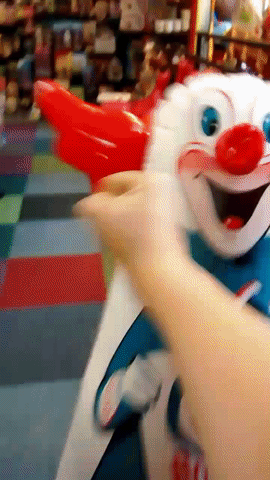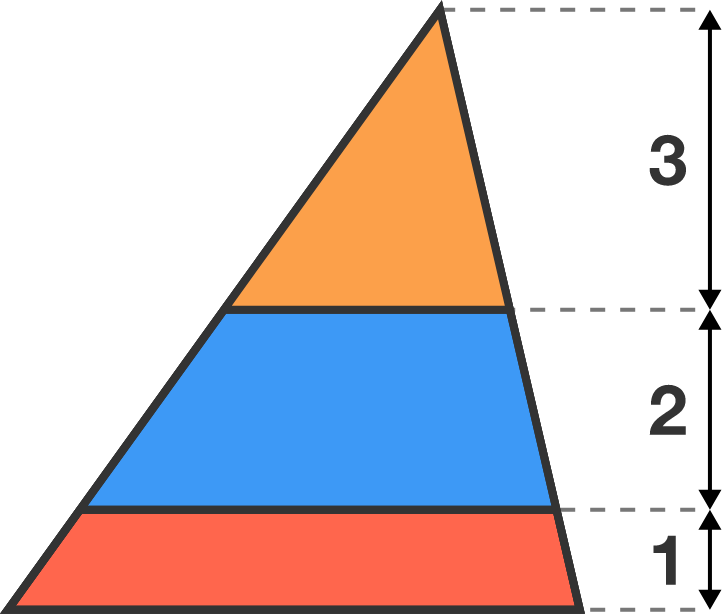The segments dividing this triangle are parallel to the triangle's base.

Which colored area is the largest?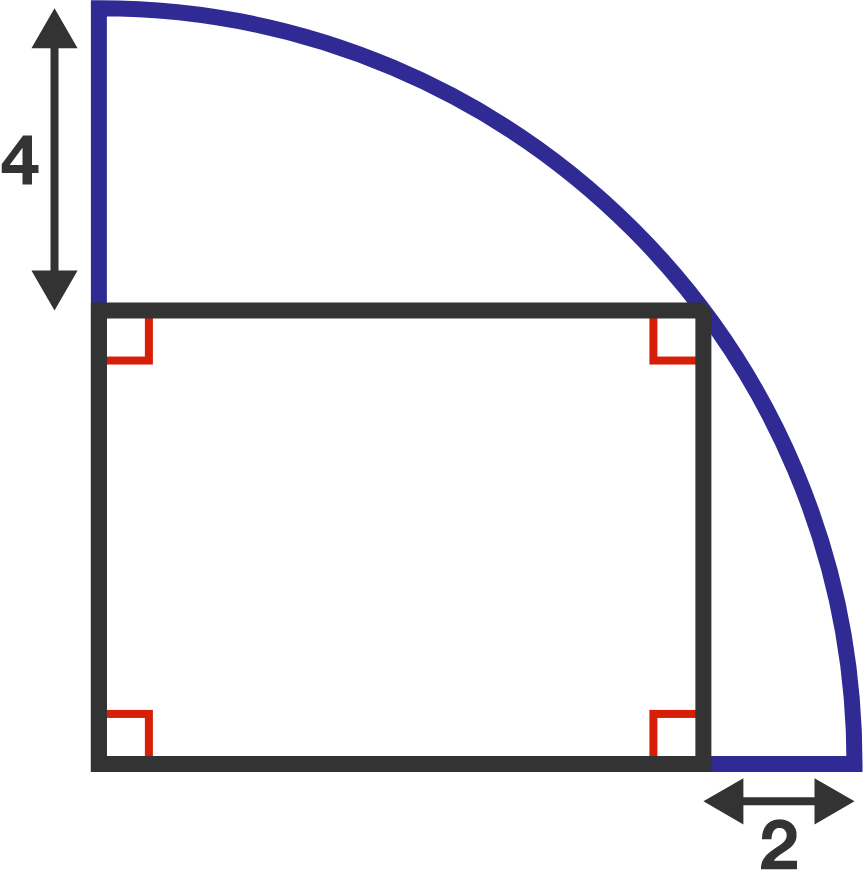What is the radius of this quarter-circle?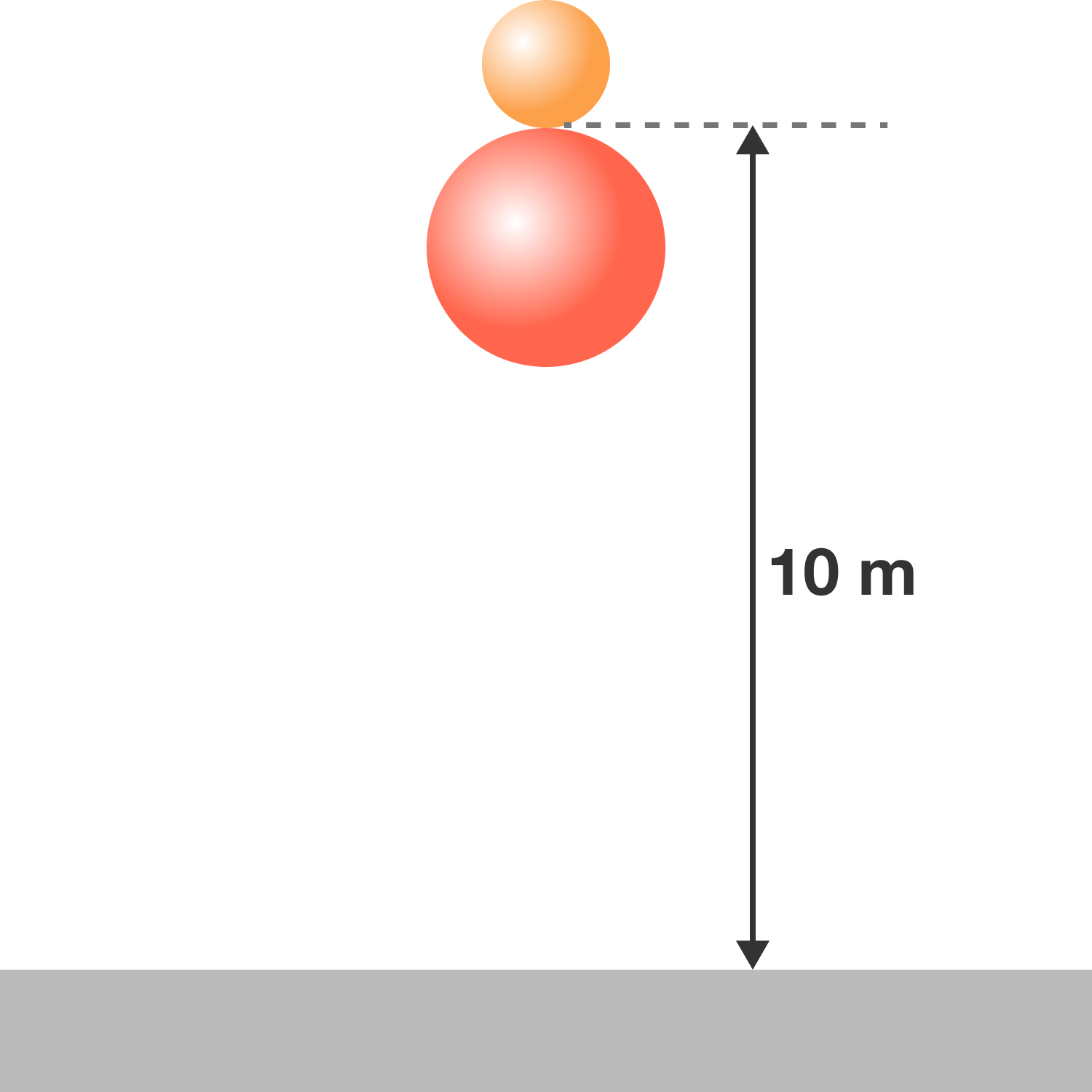A small ball is placed on top of a bigger, heavier ball at a height of $10 \text{ m}$ above the ground.

When the balls are simultaneously released and bounce, how high will the smaller ball bounce up to?

Assumptions:

• All collisions are perfectly elastic, which means no kinetic energy is lost in the collisions.
• Air resistance is negligible.

Which of the following figures has the greatest area shaded in red?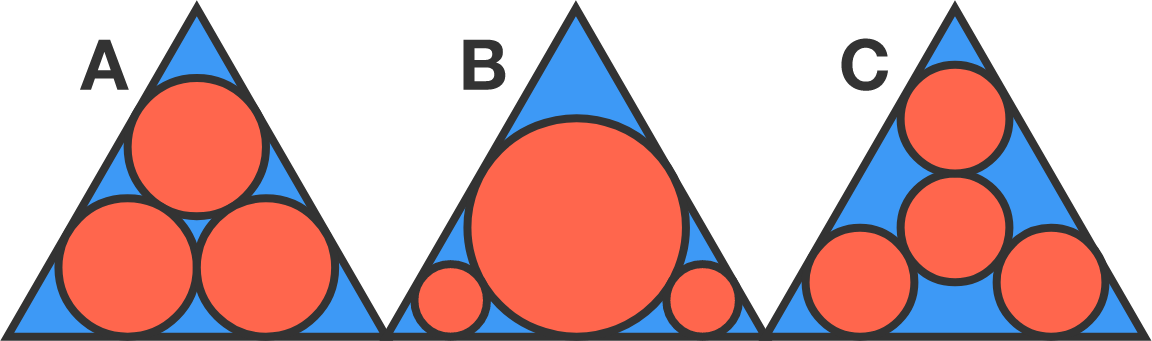Assumptions:

• A, B, C are identical equilateral triangles. The circles of A are all congruent to each other, as are the circles of C.
• The red components are circles tangent to one another and to the triangle.

Note: If you get it wrong, don't worry! An Italian mathematician named Malfatti also got it wrong in the $19^\text{th}$ century.

×